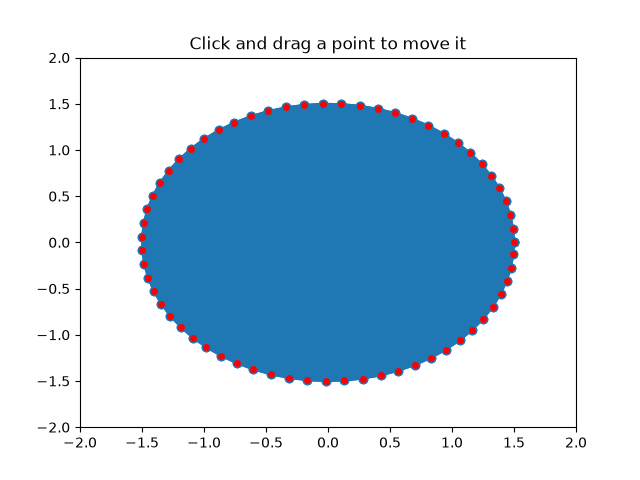# Poly Editor#

This is an example to show how to build cross-GUI applications using Matplotlib event handling to interact with objects on the canvas.

Note

This example exercises the interactive capabilities of Matplotlib, and this will not appear in the static documentation. Please run this code on your machine to see the interactivity.

You can copy and paste individual parts, or download the entire example using the link at the bottom of the page.import numpy as np

from matplotlib.artist import Artist
from matplotlib.lines import Line2D

def dist_point_to_segment(p, s0, s1):
"""
Get the distance from the point *p* to the segment (*s0*, *s1*), where
*p*, *s0*, *s1* are [x, y] arrays.
"""
s01 = s1 - s0
s0p = p - s0
if (s01 == 0).all():
return np.hypot(*s0p)
# Project onto segment, without going past segment ends.
p1 = s0 + np.clip((s0p @ s01) / (s01 @ s01), 0, 1) * s01
return np.hypot(*(p - p1))

class PolygonInteractor:
"""
A polygon editor.

Key-bindings

't' toggle vertex markers on and off.  When vertex markers are on,
you can move them, delete them

'd' delete the vertex under point

'i' insert a vertex at point.  You must be within epsilon of the
line connecting two existing vertices

"""

showverts = True
epsilon = 5  # max pixel distance to count as a vertex hit

def __init__(self, ax, poly):
if poly.figure is None:
raise RuntimeError('You must first add the polygon to a figure '
'or canvas before defining the interactor')
self.ax = ax
canvas = poly.figure.canvas
self.poly = poly

x, y = zip(*self.poly.xy)
self.line = Line2D(x, y,
marker='o', markerfacecolor='r',
animated=True)

self._ind = None  # the active vert

canvas.mpl_connect('draw_event', self.on_draw)
canvas.mpl_connect('button_press_event', self.on_button_press)
canvas.mpl_connect('key_press_event', self.on_key_press)
canvas.mpl_connect('button_release_event', self.on_button_release)
canvas.mpl_connect('motion_notify_event', self.on_mouse_move)
self.canvas = canvas

def on_draw(self, event):
self.background = self.canvas.copy_from_bbox(self.ax.bbox)
self.ax.draw_artist(self.poly)
self.ax.draw_artist(self.line)
# do not need to blit here, this will fire before the screen is
# updated

def poly_changed(self, poly):
"""This method is called whenever the pathpatch object is called."""
# only copy the artist props to the line (except visibility)
vis = self.line.get_visible()
Artist.update_from(self.line, poly)
self.line.set_visible(vis)  # don't use the poly visibility state

def get_ind_under_point(self, event):
"""
Return the index of the point closest to the event position or *None*
if no point is within self.epsilon to the event position.
"""
# display coords
xy = np.asarray(self.poly.xy)
xyt = self.poly.get_transform().transform(xy)
xt, yt = xyt[:, 0], xyt[:, 1]
d = np.hypot(xt - event.x, yt - event.y)
indseq, = np.nonzero(d == d.min())
ind = indseq

if d[ind] >= self.epsilon:
ind = None

return ind

def on_button_press(self, event):
"""Callback for mouse button presses."""
if not self.showverts:
return
if event.inaxes is None:
return
if event.button != 1:
return
self._ind = self.get_ind_under_point(event)

def on_button_release(self, event):
"""Callback for mouse button releases."""
if not self.showverts:
return
if event.button != 1:
return
self._ind = None

def on_key_press(self, event):
"""Callback for key presses."""
if not event.inaxes:
return
if event.key == 't':
self.showverts = not self.showverts
self.line.set_visible(self.showverts)
if not self.showverts:
self._ind = None
elif event.key == 'd':
ind = self.get_ind_under_point(event)
if ind is not None:
self.poly.xy = np.delete(self.poly.xy,
ind, axis=0)
self.line.set_data(zip(*self.poly.xy))
elif event.key == 'i':
xys = self.poly.get_transform().transform(self.poly.xy)
p = event.x, event.y  # display coords
for i in range(len(xys) - 1):
s0 = xys[i]
s1 = xys[i + 1]
d = dist_point_to_segment(p, s0, s1)
if d <= self.epsilon:
self.poly.xy = np.insert(
self.poly.xy, i+1,
[event.xdata, event.ydata],
axis=0)
self.line.set_data(zip(*self.poly.xy))
break
if self.line.stale:
self.canvas.draw_idle()

def on_mouse_move(self, event):
"""Callback for mouse movements."""
if not self.showverts:
return
if self._ind is None:
return
if event.inaxes is None:
return
if event.button != 1:
return
x, y = event.xdata, event.ydata

self.poly.xy[self._ind] = x, y
if self._ind == 0:
self.poly.xy[-1] = x, y
elif self._ind == len(self.poly.xy) - 1:
self.poly.xy = x, y
self.line.set_data(zip(*self.poly.xy))

self.canvas.restore_region(self.background)
self.ax.draw_artist(self.poly)
self.ax.draw_artist(self.line)
self.canvas.blit(self.ax.bbox)

if __name__ == '__main__':
import matplotlib.pyplot as plt

from matplotlib.patches import Polygon

theta = np.arange(0, 2*np.pi, 0.1)
r = 1.5

xs = r * np.cos(theta)
ys = r * np.sin(theta)

poly = Polygon(np.column_stack([xs, ys]), animated=True)

fig, ax = plt.subplots()